# 3.2.4.3.1. sklearn.ensemble.RandomForestClassifier¶

class sklearn.ensemble.RandomForestClassifier(n_estimators=’warn’, criterion=’gini’, max_depth=None, min_samples_split=2, min_samples_leaf=1, min_weight_fraction_leaf=0.0, max_features=’auto’, max_leaf_nodes=None, min_impurity_decrease=0.0, min_impurity_split=None, bootstrap=True, oob_score=False, n_jobs=None, random_state=None, verbose=0, warm_start=False, class_weight=None)[source]

A random forest classifier.

A random forest is a meta estimator that fits a number of decision tree classifiers on various sub-samples of the dataset and uses averaging to improve the predictive accuracy and control over-fitting. The sub-sample size is always the same as the original input sample size but the samples are drawn with replacement if bootstrap=True (default).

Read more in the User Guide.

Parameters: n_estimators : integer, optional (default=10) The number of trees in the forest. Changed in version 0.20: The default value of n_estimators will change from 10 in version 0.20 to 100 in version 0.22. criterion : string, optional (default=”gini”) The function to measure the quality of a split. Supported criteria are “gini” for the Gini impurity and “entropy” for the information gain. Note: this parameter is tree-specific. max_depth : integer or None, optional (default=None) The maximum depth of the tree. If None, then nodes are expanded until all leaves are pure or until all leaves contain less than min_samples_split samples. min_samples_split : int, float, optional (default=2) The minimum number of samples required to split an internal node: If int, then consider min_samples_split as the minimum number. If float, then min_samples_split is a fraction and ceil(min_samples_split * n_samples) are the minimum number of samples for each split. Changed in version 0.18: Added float values for fractions. min_samples_leaf : int, float, optional (default=1) The minimum number of samples required to be at a leaf node. A split point at any depth will only be considered if it leaves at least min_samples_leaf training samples in each of the left and right branches. This may have the effect of smoothing the model, especially in regression. If int, then consider min_samples_leaf as the minimum number. If float, then min_samples_leaf is a fraction and ceil(min_samples_leaf * n_samples) are the minimum number of samples for each node. Changed in version 0.18: Added float values for fractions. min_weight_fraction_leaf : float, optional (default=0.) The minimum weighted fraction of the sum total of weights (of all the input samples) required to be at a leaf node. Samples have equal weight when sample_weight is not provided. max_features : int, float, string or None, optional (default=”auto”) The number of features to consider when looking for the best split: If int, then consider max_features features at each split. If float, then max_features is a fraction and int(max_features * n_features) features are considered at each split. If “auto”, then max_features=sqrt(n_features). If “sqrt”, then max_features=sqrt(n_features) (same as “auto”). If “log2”, then max_features=log2(n_features). If None, then max_features=n_features. Note: the search for a split does not stop until at least one valid partition of the node samples is found, even if it requires to effectively inspect more than max_features features. max_leaf_nodes : int or None, optional (default=None) Grow trees with max_leaf_nodes in best-first fashion. Best nodes are defined as relative reduction in impurity. If None then unlimited number of leaf nodes. min_impurity_decrease : float, optional (default=0.) A node will be split if this split induces a decrease of the impurity greater than or equal to this value. The weighted impurity decrease equation is the following: N_t / N * (impurity - N_t_R / N_t * right_impurity - N_t_L / N_t * left_impurity)  where N is the total number of samples, N_t is the number of samples at the current node, N_t_L is the number of samples in the left child, and N_t_R is the number of samples in the right child. N, N_t, N_t_R and N_t_L all refer to the weighted sum, if sample_weight is passed. New in version 0.19. min_impurity_split : float, (default=1e-7) Threshold for early stopping in tree growth. A node will split if its impurity is above the threshold, otherwise it is a leaf. Deprecated since version 0.19: min_impurity_split has been deprecated in favor of min_impurity_decrease in 0.19. The default value of min_impurity_split will change from 1e-7 to 0 in 0.23 and it will be removed in 0.25. Use min_impurity_decrease instead. bootstrap : boolean, optional (default=True) Whether bootstrap samples are used when building trees. If False, the whole datset is used to build each tree. oob_score : bool (default=False) Whether to use out-of-bag samples to estimate the generalization accuracy. n_jobs : int or None, optional (default=None) The number of jobs to run in parallel for both fit and predict. None means 1 unless in a joblib.parallel_backend context. -1 means using all processors. See Glossary for more details. random_state : int, RandomState instance or None, optional (default=None) If int, random_state is the seed used by the random number generator; If RandomState instance, random_state is the random number generator; If None, the random number generator is the RandomState instance used by np.random. verbose : int, optional (default=0) Controls the verbosity when fitting and predicting. warm_start : bool, optional (default=False) When set to True, reuse the solution of the previous call to fit and add more estimators to the ensemble, otherwise, just fit a whole new forest. See the Glossary. class_weight : dict, list of dicts, “balanced”, “balanced_subsample” or None, optional (default=None) Weights associated with classes in the form {class_label: weight}. If not given, all classes are supposed to have weight one. For multi-output problems, a list of dicts can be provided in the same order as the columns of y. Note that for multioutput (including multilabel) weights should be defined for each class of every column in its own dict. For example, for four-class multilabel classification weights should be [{0: 1, 1: 1}, {0: 1, 1: 5}, {0: 1, 1: 1}, {0: 1, 1: 1}] instead of [{1:1}, {2:5}, {3:1}, {4:1}]. The “balanced” mode uses the values of y to automatically adjust weights inversely proportional to class frequencies in the input data as n_samples / (n_classes * np.bincount(y)) The “balanced_subsample” mode is the same as “balanced” except that weights are computed based on the bootstrap sample for every tree grown. For multi-output, the weights of each column of y will be multiplied. Note that these weights will be multiplied with sample_weight (passed through the fit method) if sample_weight is specified. estimators_ : list of DecisionTreeClassifier The collection of fitted sub-estimators. classes_ : array of shape = [n_classes] or a list of such arrays The classes labels (single output problem), or a list of arrays of class labels (multi-output problem). n_classes_ : int or list The number of classes (single output problem), or a list containing the number of classes for each output (multi-output problem). n_features_ : int The number of features when fit is performed. n_outputs_ : int The number of outputs when fit is performed. feature_importances_ : array of shape = [n_features] Return the feature importances (the higher, the more important the feature). oob_score_ : float Score of the training dataset obtained using an out-of-bag estimate. oob_decision_function_ : array of shape = [n_samples, n_classes] Decision function computed with out-of-bag estimate on the training set. If n_estimators is small it might be possible that a data point was never left out during the bootstrap. In this case, oob_decision_function_ might contain NaN.

DecisionTreeClassifier, ExtraTreesClassifier

Notes

The default values for the parameters controlling the size of the trees (e.g. max_depth, min_samples_leaf, etc.) lead to fully grown and unpruned trees which can potentially be very large on some data sets. To reduce memory consumption, the complexity and size of the trees should be controlled by setting those parameter values.

The features are always randomly permuted at each split. Therefore, the best found split may vary, even with the same training data, max_features=n_features and bootstrap=False, if the improvement of the criterion is identical for several splits enumerated during the search of the best split. To obtain a deterministic behaviour during fitting, random_state has to be fixed.

References

 [R45f14345c000-1] Breiman, “Random Forests”, Machine Learning, 45(1), 5-32, 2001.

Examples

>>> from sklearn.ensemble import RandomForestClassifier
>>> from sklearn.datasets import make_classification

>>> X, y = make_classification(n_samples=1000, n_features=4,
...                            n_informative=2, n_redundant=0,
...                            random_state=0, shuffle=False)
>>> clf = RandomForestClassifier(n_estimators=100, max_depth=2,
...                              random_state=0)
>>> clf.fit(X, y)
RandomForestClassifier(bootstrap=True, class_weight=None, criterion='gini',
max_depth=2, max_features='auto', max_leaf_nodes=None,
min_impurity_decrease=0.0, min_impurity_split=None,
min_samples_leaf=1, min_samples_split=2,
min_weight_fraction_leaf=0.0, n_estimators=100, n_jobs=None,
oob_score=False, random_state=0, verbose=0, warm_start=False)
>>> print(clf.feature_importances_)
[0.14205973 0.76664038 0.0282433  0.06305659]
>>> print(clf.predict([[0, 0, 0, 0]]))



Methods

 apply(self, X) Apply trees in the forest to X, return leaf indices. decision_path(self, X) Return the decision path in the forest fit(self, X, y[, sample_weight]) Build a forest of trees from the training set (X, y). get_params(self[, deep]) Get parameters for this estimator. predict(self, X) Predict class for X. predict_log_proba(self, X) Predict class log-probabilities for X. predict_proba(self, X) Predict class probabilities for X. score(self, X, y[, sample_weight]) Returns the mean accuracy on the given test data and labels. set_params(self, \*\*params) Set the parameters of this estimator.
__init__(self, n_estimators=’warn’, criterion=’gini’, max_depth=None, min_samples_split=2, min_samples_leaf=1, min_weight_fraction_leaf=0.0, max_features=’auto’, max_leaf_nodes=None, min_impurity_decrease=0.0, min_impurity_split=None, bootstrap=True, oob_score=False, n_jobs=None, random_state=None, verbose=0, warm_start=False, class_weight=None)[source]
apply(self, X)[source]

Apply trees in the forest to X, return leaf indices.

Parameters: X : array-like or sparse matrix, shape = [n_samples, n_features] The input samples. Internally, its dtype will be converted to dtype=np.float32. If a sparse matrix is provided, it will be converted into a sparse csr_matrix. X_leaves : array_like, shape = [n_samples, n_estimators] For each datapoint x in X and for each tree in the forest, return the index of the leaf x ends up in.
decision_path(self, X)[source]

Return the decision path in the forest

New in version 0.18.

Parameters: X : array-like or sparse matrix, shape = [n_samples, n_features] The input samples. Internally, its dtype will be converted to dtype=np.float32. If a sparse matrix is provided, it will be converted into a sparse csr_matrix. indicator : sparse csr array, shape = [n_samples, n_nodes] Return a node indicator matrix where non zero elements indicates that the samples goes through the nodes. n_nodes_ptr : array of size (n_estimators + 1, ) The columns from indicator[n_nodes_ptr[i]:n_nodes_ptr[i+1]] gives the indicator value for the i-th estimator.
feature_importances_
Return the feature importances (the higher, the more important the
feature).
Returns: feature_importances_ : array, shape = [n_features] The values of this array sum to 1, unless all trees are single node trees consisting of only the root node, in which case it will be an array of zeros.
fit(self, X, y, sample_weight=None)[source]

Build a forest of trees from the training set (X, y).

Parameters: X : array-like or sparse matrix of shape = [n_samples, n_features] The training input samples. Internally, its dtype will be converted to dtype=np.float32. If a sparse matrix is provided, it will be converted into a sparse csc_matrix. y : array-like, shape = [n_samples] or [n_samples, n_outputs] The target values (class labels in classification, real numbers in regression). sample_weight : array-like, shape = [n_samples] or None Sample weights. If None, then samples are equally weighted. Splits that would create child nodes with net zero or negative weight are ignored while searching for a split in each node. In the case of classification, splits are also ignored if they would result in any single class carrying a negative weight in either child node. self : object
get_params(self, deep=True)[source]

Get parameters for this estimator.

Parameters: deep : boolean, optional If True, will return the parameters for this estimator and contained subobjects that are estimators. params : mapping of string to any Parameter names mapped to their values.
predict(self, X)[source]

Predict class for X.

The predicted class of an input sample is a vote by the trees in the forest, weighted by their probability estimates. That is, the predicted class is the one with highest mean probability estimate across the trees.

Parameters: X : array-like or sparse matrix of shape = [n_samples, n_features] The input samples. Internally, its dtype will be converted to dtype=np.float32. If a sparse matrix is provided, it will be converted into a sparse csr_matrix. y : array of shape = [n_samples] or [n_samples, n_outputs] The predicted classes.
predict_log_proba(self, X)[source]

Predict class log-probabilities for X.

The predicted class log-probabilities of an input sample is computed as the log of the mean predicted class probabilities of the trees in the forest.

Parameters: X : array-like or sparse matrix of shape = [n_samples, n_features] The input samples. Internally, its dtype will be converted to dtype=np.float32. If a sparse matrix is provided, it will be converted into a sparse csr_matrix. p : array of shape = [n_samples, n_classes], or a list of n_outputs such arrays if n_outputs > 1. The class probabilities of the input samples. The order of the classes corresponds to that in the attribute classes_.
predict_proba(self, X)[source]

Predict class probabilities for X.

The predicted class probabilities of an input sample are computed as the mean predicted class probabilities of the trees in the forest. The class probability of a single tree is the fraction of samples of the same class in a leaf.

Parameters: X : array-like or sparse matrix of shape = [n_samples, n_features] The input samples. Internally, its dtype will be converted to dtype=np.float32. If a sparse matrix is provided, it will be converted into a sparse csr_matrix. p : array of shape = [n_samples, n_classes], or a list of n_outputs such arrays if n_outputs > 1. The class probabilities of the input samples. The order of the classes corresponds to that in the attribute classes_.
score(self, X, y, sample_weight=None)[source]

Returns the mean accuracy on the given test data and labels.

In multi-label classification, this is the subset accuracy which is a harsh metric since you require for each sample that each label set be correctly predicted.

Parameters: X : array-like, shape = (n_samples, n_features) Test samples. y : array-like, shape = (n_samples) or (n_samples, n_outputs) True labels for X. sample_weight : array-like, shape = [n_samples], optional Sample weights. score : float Mean accuracy of self.predict(X) wrt. y.
set_params(self, **params)[source]

Set the parameters of this estimator.

The method works on simple estimators as well as on nested objects (such as pipelines). The latter have parameters of the form <component>__<parameter> so that it’s possible to update each component of a nested object.

Returns: self

## 3.2.4.3.1.1. Examples using sklearn.ensemble.RandomForestClassifier¶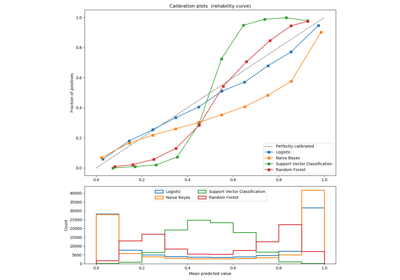Comparison of Calibration of Classifiers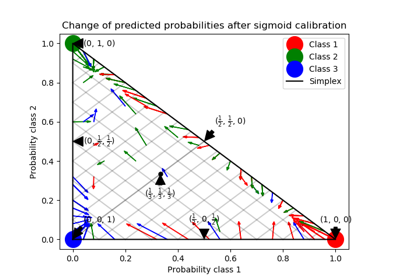Probability Calibration for 3-class classification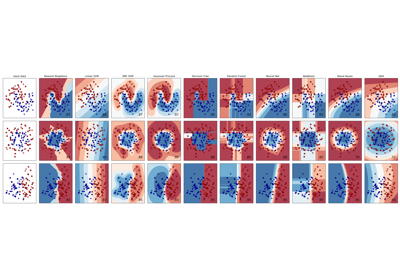Classifier comparison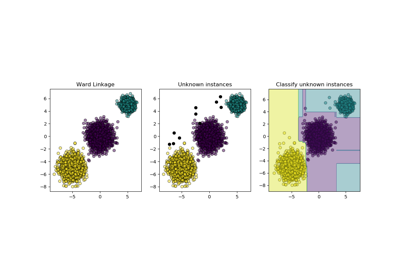Inductive Clustering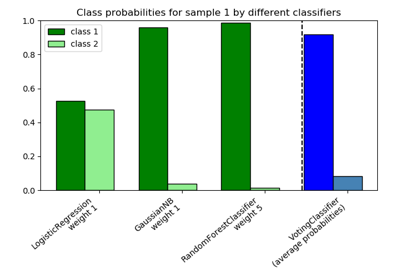Plot class probabilities calculated by the VotingClassifier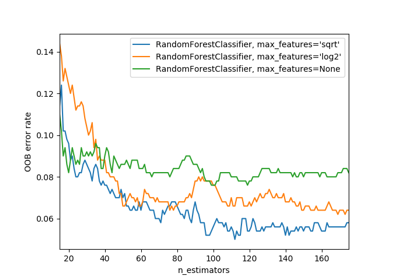OOB Errors for Random Forests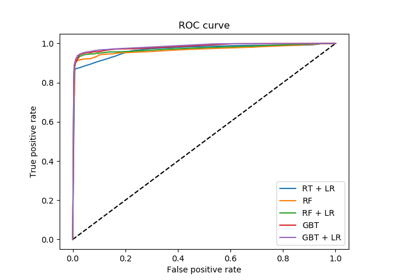Feature transformations with ensembles of trees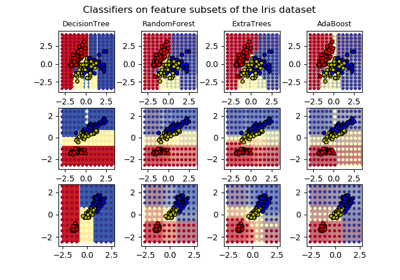Plot the decision surfaces of ensembles of trees on the iris datasetComparing randomized search and grid search for hyperparameter estimation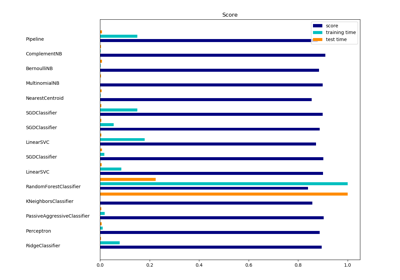Classification of text documents using sparse features